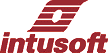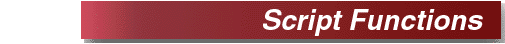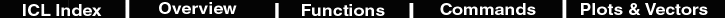Mathematical functions Single argument
```
mag,magnitudeReturns the magnitude of a complex vector in a real vector
ph,phaseReturns the phase of a complex vector in a real vector
jsqrt(-1) * vec
re,realPlace the real part of a complex vector in a real vector
im,imagPlace the imaginary part of a complex vector in a real vector
db20 * lgt(vec)
log,log10Log base 10 of vec
lnNatural logisdeftest existence of a vector; returns 1 if it exists, 0 otherwise
expe ^ vec
absAbsolute value
sqrtSquare rootderivx,differentiatex(Scope  Only) Returns the derivative of a vector with respect tothe default scale vector (usually time or frequency).
deriv,differentiateReturns the derivative of a vector with respect to the current plots scale
ceilReturns the ceiling of the vector.
floorReturns the floor of the vector.integratex(Scope Only) Returns the integral of a vector with respect to thedefault scale vector (usually  time or frequency).
integrateReturns the integral of a vector with respect to the current plots scale
pulseReturns a vector that is the length of the default vector that is unity forthe number of points in its argument and zero thereafter```
`Trigonometric functions`
```
sincostanatan```
```Miscellaneous functions
Single argument except as noted```
```askvalueor Scope5 only) Displays a dialog box asking the user to enter a number(real or a complex) which is returned by the function when theuser clicks OK. The argument to the function is the prompt stringto be displayed in the dialog.isdisplayed(for Scope5 only) Return 1if the argument is the name of a vector that is currentlydisplayed as a trace in Scope, otherwise 0. If the argument is asimple vector name, the currently active plot is searched. If theargument is a plot name, vector name combination of the form<plotName>.<vecName>, the specified plot is searched.
normNormalize the vector so the max of its abs is 1.0
rndRandom integer between 0 and vec.
posReturn 1 if vec[] > 0, else 0.
vectorReturn a vector from 0 to the magnitude of the argument, wherelength is the argument value caste to an integer.
unitvecReturns a vector composed of all 1’s whose length is the argument.
lengthReturns the length of the vector argument.
interpolateRe-scales a vector from another plot to the current plot.
phaseextendExtends phase past +-180 degrees, assumes the initial phase iswithin the +-180 degree boundary.
operatingpointReturns the magnitude of the first element.
getcursorxReturns the value of the cursor (x-axis scale).
getcursoryFunction with 2 arguments, vec and cursor number returns the valueof the vector identified by cursor number.
getcursory0(v(1))Returns the vector value corresponding to cursor 0 for vector v(1).
getcursory1(v(1))Returns the vector value corresponding to cursor 1 for vector v(1).
initialvalueThe initial value of a vector.
isdefReturns 0 if the argument is not a vector, else returns 1. finalvalueThe last value of a vector. nextvectorEnumerates a vector list, the first vector is returned if the argumentis null, null is returned at the end. The return value is an alias to thevector. If you use the plot resolution operator, refplot.nv, then you willenumerate the refplot vectors.
nextplotEnumerates a plot list, the first plots vector is returned if the argumentis null, null is returned at the end. The return value is an alias to the plotscale, do not use plot the resulution operator, plot.pl, for this vector.
sameplotReturns 1 if a vector is in the current plot.
Cursor relative functions,
Evaluate the vector between cursor 0 and cursor 1
single argument```
```
rmsRoot Mean Square by trapezoid integrationrmsptsRoot Mean Square by datapoints
mean,averageAverage value by trapezoid integrationmeanptsAverage value by datapointsmaxMaximum valueminMinimum valuemaxscaleMaximum scale valueminscaleMinimum scale value

stddevStandard deviation (rms with average removed) by trapezoid integration
stddevptsStandard deviation by datapointspk_pkPeak to peak
triseThe 10-90% transition using cursor 0 and cursor 1 to define initial and final value.
tfallThe 10-90% transition using cursor 0 and cursor 1 to define initial and final value.```
Operations
Between 2 values
 Operation Symbol Example PLUS "+" x = a + b MINUS "-" x = a - b TIMES "*" x = a * b MOD "%" x = a % b - remainder of integer division DIVIDE "/" x = a/b COMMA "," x = vreal, vimaginary POWER "^" xsquared = x^2 EQ "=" if a = b GT ">" if a > b LT "<" if a < b GE ">=" if a >= b LE "<=" if a <= b NE "<>" if a <> b AND "&" vand = a & b OR "|" vor = a | b INDX "[" vthree = a UMINUS, "-" x = -b NOT "~" vnand = ~vand

Assignment operators that extract the mid part of a vector

 vmid=w1[(20,30)] It makes a new vector, vmid, using elements 20 through 30 of vector w1. vmid=w1[[(500u,600u)]] Extracts data based on the scale vector range (time in this case) and makes a new vector out of the data between 500u to 600u. You can make an accompanying time axis for a new plot: t56 = time[[(500u,600u)]], and then plot vmid vs t56. Of course you can subtract the offset from t56 using the following script: t56 = t56 - 500u. This gives you the power to rearrange data during post processing to make things like "eye" diagrams.